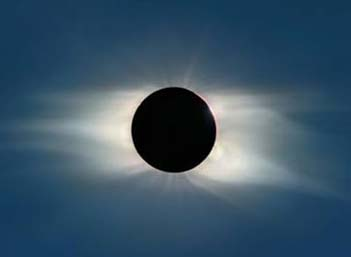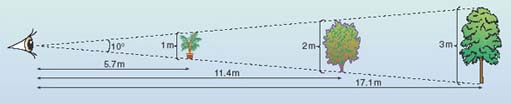The Sun

This free course is available to start right now. Review the full course description and key learning outcomes and create an account and enrol if you want a free statement of participation.

Free course

# 3.1 Angular sizeFigure 19 A total eclipse of the Sun, revealing the outer part of the Sun's atmosphere, the corona, and the inner part, the chromosphere, which can just be seen as a reddish tinge on the upper right limb.

The image shown in Figure 1 at the start of this course (repeated above), was taken during a total eclipse of the Sun, in which the Moon blocked out light from the Sun's photosphere, enabling the chromosphere and the corona to be seen. This happens because of a remarkable coincidence. The Sun is very much bigger than the Moon - about 400 times bigger in diameter - but it is also very much further away, by almost exactly the same factor. This means that the Sun and the Moon appear the same size in the sky: that is, the Sun and the Moon have the same angular size. Figure 20 illustrates this idea by showing lines drawn from an observer's eye to the extreme edges of objects at various distances. The angle between the lines determines the angular size of the objects, which would be 10° in all three cases, according to the shown observer. Angular size thus depends on an object's actual size and its distance from the observer's eye.Figure 20 The observed angular size of an object depends on the size of the object and its distance from the observer.

You are probably familiar with angles measured in degrees (360° for a full circle, 90° for a right angle, and so on). However, although the objects studied by astronomers are very large, they are at such vast distances that their angular sizes are often very small. These small angles could be written as fractions of a degree or their decimal equivalents but, in practice, subdivisions of degrees are used, known as minutes of arc (or arcmin) and seconds of arc (or arcsec). A degree can be divided into 60 minutes of arc, and a minute of arc can be further divided into 60 seconds of arc. A single tick mark is used to represent arcmin, so 1/60 of a degree is written 1′. A double tick mark denotes arcsec, so 1/3600 of a degree (1/60 of an arcmin) is written 1″.

Angular size is a very useful quantity in astronomy, since angular sizes are very much easier to measure than actual sizes because they refer simply to how large an object appears in the sky. The Sun and the Moon both have an angular size of about half a degree, which is about 30 arcmin or 30′.

## Question 5

• How would you define the angular size of the Moon?

It is the angle between two imaginary lines drawn from an observer's eye to the extremities of the disc of the Moon. (More formally, it is the angle subtended at the eye of the observer by the diameter of the Moon.)

S194_1